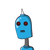# 2.The value of x, if 3, 8, x, 42 are in proportion is:(a) 7 (b) 9(c) 64 (d) none of these​

2.The value of x, if 3, 8, x, 42 are in proportion is:
(a) 7 (b) 9
(c) 64 (d) none of these​

### 1 thought on “2.The value of x, if 3, 8, x, 42 are in proportion is:<br />(a) 7 (b) 9<br />(c) 64 (d) none of these​”

1.(d) none of these

Step-by-step explanation:

3/8 = x/42

3 X 42 = 8 X x

126 = 8x

x = 15.7

therefore, none of these answers – d